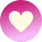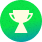Search Community

# Snap element to path

## Recommended Posts

Hi

I have a wiggly path with circles that should match the Y position of some texts, and the X position of a path.

I tried using MotionPath and calculate the "progress", but it won't be accurate as the path is wiggly.

Is there some way to attach the circle to the path, but only for X axis? I got the Y axis down, so that's out of scope of this question.

I guess another way of doing it is to convert known Y, to a progress on a path. Is that possible?

##### Share on other sites

• blueblau changed the title to Snap element to path

Hi @blueblau. Are you trying to basically map the position on the curve (non-linear) to the scroll position (linear)? I've been curious about this for a while and I had an idea, so I spent all day developing a helper function. The concept is to craft a custom ease that bends time such that it approximates matching up with the overall progress of the path animation on the y axis. So if you set up your ScrollTrigger properly (basically make sure the end position is exactly the same distance from the start as the path is tall which you could do like `end: () => "+=" + document.querySelector("#motionPath").getBoundingClientRect().height`) then it should keep whatever is traveling on the motion path pretty well at the same spot vertically in the viewport as you scroll. Fun!

It was not a trivial thing (which is why it took me all day), but I think it turned out to be relatively effective:

```// helper function to adjust the ease so that the tractor stays relatively centered. Requires MotionPathPlugin of course
function pathEase(path, axis="y", precision=1) {
let rawPath = MotionPathPlugin.cacheRawPathMeasurements(MotionPathPlugin.getRawPath(gsap.utils.toArray(path)), Math.round(precision * 12)),
useX = axis === "x",
start = rawPath[useX ? 0 : 1],
end = rawPath[rawPath.length - 1][rawPath[rawPath.length-1].length - (useX ? 2 : 1)],
range = end - start,
l = Math.round(precision * 200),
inc = 1 / l,
positions = ,
a = [],
minIndex = 0,
getClosest = p => {
while (positions[minIndex] <= p && minIndex++ < l) { }
a.push((p - positions[minIndex-1]) / (positions[minIndex] - positions[minIndex - 1]) * inc + minIndex * inc);
},
i = 1,
p, v;
for (; i < l; i++) {
p = i / l;
v = MotionPathPlugin.getPositionOnPath(rawPath, p)[axis];
positions[i] = (v - start) / range;
}
positions[l] = 1;
for (i = 0; i < l; i++) {
getClosest(i / l);
}
a.push(1);
return p => {
let i = p * l,
s = a[i | 0];
return i ? s + (a[Math.ceil(i)] - s) * (i % 1) : 0;
}
}```

Usage:

`ease: pathEase("#motionPath")`

I tried it on this Demo:

See the Pen GRoXzYj?editors=0010 by GreenSock (@GreenSock) on CodePen

Notice if you change the ease to `"none"` (or anything else), the tractor position in the viewport vertically varies widely and can even be out of view in some cases. But not with the magic of the pathEase() helper functionLet me know if that helps.

•1
•1
##### Share on other sites

Hi, thank you for helping out.

It's just a static presentation, so I actually ended up looping through the path length and finding the point on the path where Y were closest to the Y of the headline, and the setting the circles to that position with gsap.set.

There are tradeoffs to this implementation. I can't transition them along the path to this position, for one.

I just tried your solution (which of course seems like the right way to do it), on a old version of my codebase. However the positions were unchanged after applying the ease-function. I did things a bit differently than the tractor demo, maybe that's why I can't get it to work. I positioned the Circle with MotionPath by using the `end` parameter as a progress. The progress was calculated by mapping the headlines position relative to their parent, to a normalized 0-1 value.

##### Share on other sites

Ah, okay. Another fun challenge for me! 🥳

Here's a helper function that lets you feed in a target element (like your headline) and a motion path and it'll spit back the progress value (between 0 and 1) corresponding to where it'll hit the center of that target element on the given axis ("y" axis by default):

```// helper function that returns the progress value for a motion path where it hits the center of the provided target on the given axis ("y" by default).
function findProgress(target, path, {axis="y", precision=1, ease="none"}={}) {
target = gsap.utils.toArray(target);
path = gsap.utils.toArray(path);
ease = gsap.parseEase(ease) || (p => p);
let tBounds = target.getBoundingClientRect(),
pBounds = path.getBoundingClientRect(),
useX = axis === "x",
tCenter = (tBounds[useX ? "left" : "top"] + tBounds[useX ? "right" : "bottom"]) / 2,
rawPath = MotionPathPlugin.cacheRawPathMeasurements(MotionPathPlugin.getRawPath(path), Math.round(precision * 12)),
start = rawPath[useX ? 0 : 1],
end = rawPath[rawPath.length - 1][rawPath[rawPath.length-1].length - (useX ? 2 : 1)],
pinpoint = gsap.utils.mapRange(pBounds[useX ? "left" : "top"], pBounds[useX ? "right" : "bottom"], start, end, tCenter),
l = Math.round(precision * 200),
inc = 1 / l,
i = 1,
prevV = start,
p, v;
if (pinpoint < Math.min(start, end)) {
p = start < end ? 0 : 1;
} else if (pinpoint > Math.max(start, end)) {
p = start < end ? 1 : 0;
} else {
for (; i < l; i++) {
p = i / l;
v = MotionPathPlugin.getPositionOnPath(rawPath, ease(p))[axis];
if ((v >= pinpoint && prevV < pinpoint) || (v <= pinpoint && prevV > pinpoint)) {
return p - (1 - gsap.utils.normalize(prevV, v, pinpoint)) * inc;
}
prevV = v;
}
}
return p;
}```

Here's a demo where I just placed a horizontal blue bar 1300px from the top (change the CSS to whatever you want) and it'll set the progress of the motionPath so that the tractor is right at that spot:

See the Pen BaPdrKM?editors=0110 by GreenSock (@GreenSock) on CodePen

If it's not clear, please provide a minimal demo (like a CodePen) with your setup simplified as much as possible, and I don't mind wiring it up for you.

I hope this helps you (or someone else)•4
•1
##### Share on other sites

Ah great. I will try to add this tomorrow, when I'm back at the office.

Thanks! 💪

Will get back to you to know how it went.

##### Share on other sites

Wow @GreenSock this is incredible.

•1